Subsections

# Thermodynamic Properties in NVT Ensemble

## Pressure.

As we pointed out in the beginning of this chapter, the main purpose of the computer simulations is to calculate thermodynamic properties. They are calculated as averages of the corresponding functions that depend on the particle coordinates. The averaging is done over the configurations, produced during the MC run.

For many quantities, like magnetization or energy, the calculations are obvious. Pressure is a little more tricky, and now we are going to derive the expression for it.

As we remember from the thermodynamics: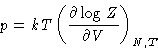where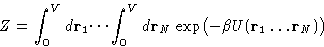For simplicity, we assume, that the simulation cell has cubic shape, and make the coordinate change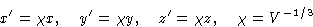Then the coordinate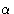of the particle i is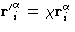, and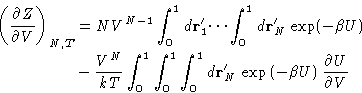(1)
with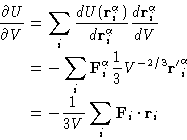(2)

Now after going back to the initial coordinates and substituting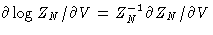, we see, that the first term is just density, and the second is an ensemble average of the sum of the force times coordinate.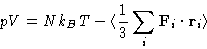The second term on the right hand side is called virial, and this equation is often called the virial equation. If the interaction potential is pairwise, it can be rearranged as follows: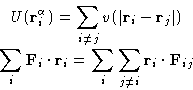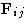is the force, acting on the atom i from the atom j.(Why?).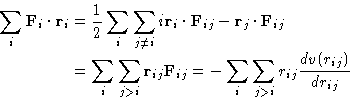(3)

Finally, we obtain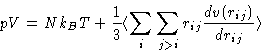(4)
Equation 4 should look familiar. Before, when we discussed the theory of liquids , we derived the same expression for pressure but in terms of the pairwise interaction potential and g(r). Here we derived more general expression, applicable even in the case when the interactions are not necessarily pairwise. It also does not require calculations of g(r), that can be very involved.

## Chemical potential

If we have a system of N particles at temperature T, and volume V, the chemical potentialat large enough N can be written as: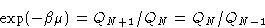where QN is partition function for the system of N particles in the volume N. The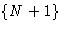-particle partition function is: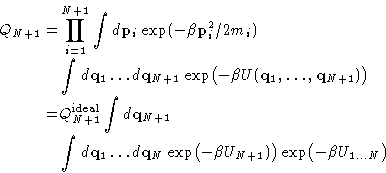(5)
Here we split the total system energy into the interaction UN+1 of the N+1-st particle with the other N particles, and the energy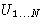of the N-particle system. The integral in the last equation can be rewritten as the ensemble average of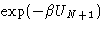over the ensemble of systems with N particles: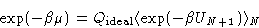This is the basis for the so-called
Widom test-particle method.
• Perform NVT MC simulation for the system of N particles
• Calculate the average of the, by inserting the ghost'' particle at random positions inside the box, and calculating its interaction energy with the rest of the system, as if it were real.
This technique works fine at moderate densities around the critical point, but turns out to have problems at high densities around liquid-solid coexistence, because the probability to insert the ghost'' at the place where the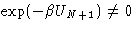is very small.

## Pair distribution function g(r)

The importance of the pair distribution function g(r) was discussed in the chapter on the liquid-state theory . Its calculation in the MC simulations is really straightforward. All we have to do is just to count the number of particles at the distances between r and r+dr from the chosen particle. In the homogeneous systems it is also averaged over all particles. It is calculated as a histogram with the bin width dr, and then is normalized by the area of the spherical layer.

Code example
(to be added to the MC code). Notice, that it is 2D! Code.

## Long-Range Corrections

In the previous lecture we mentioned, that it is very convenient to cut the interaction potential after certain cut-off distance rc. The thermodynamics properties has to be corrected for this approximation. The expressions are listed below: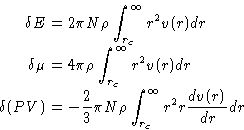(6)Next: NPT Ensemble Up: Calculation of Thermodynamic Properties. Previous: Calculation of Thermodynamic Properties.

© 1997 Boris Veytsman and Michael Kotelyanskii
Thu Nov 13 19:07:05 EST 1997